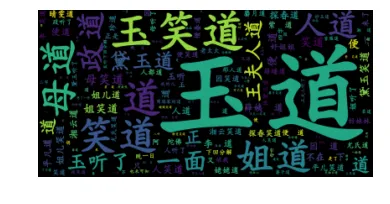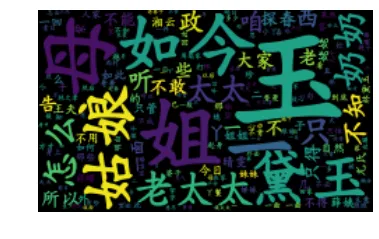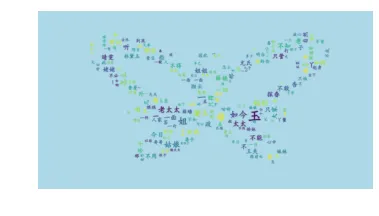# 2.绘制《红楼梦》词云

    from wordcloud import WordCloud
import jieba
wordcloud = WordCloud(font_path="kaibold.ttf").generate(text)

# Display the generated image:
plt.imshow(wordcloud, interpolation='bilinear')
plt.axis("off")
plt.margins(x=0, y=0)
plt.show()# 3.特定图形的词云

    from wordcloud import WordCloud
import jieba,requests
from PIL import Image
import numpy as np
remove_word = [i.strip() for i in open("remove.txt").readlines()]
for i in remove_word:
text = text.replace(i+" ","")

# Make the figure

plt.imshow(wordcloud, interpolation='bilinear')
plt.axis("off")
plt.margins(x=0, y=0)
plt.show()



这里使用本站绘制的蝴蝶曲线生成的词云图形，如下